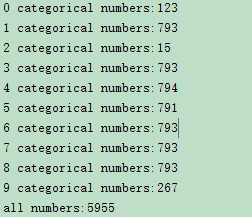# Why is the validation set highly accurate , and the test set is only 2%, and the weighted loss is about 30% (acc is low)?

(cuixing) #1

When I classify digital images for identification (sample distribution is not balanced)，why is the validation set highly accurate , and the test set is only 2%, and the weighted loss is about 30% (accuracy is low)?
Is there any other way to improve the accuracy of the test set?

The distribution of my training set is:all code is here:

``````# -*- coding: utf-8 -*-
"""
Created on Fri May 10 09:03:47 2019

# https://ww2.mathworks.cn/matlabcentral/answers/461076-why-is-the-validation-set-highly-accurate-99-and-the-test-set-is-only-21-and-the-weighted-loss
@author: cuixingxing
"""

import torch
import torch.nn as nn
from torch.utils.data import DataLoader
import torchvision
import torchvision.transforms as transforms
import torch.nn.functional as F
import time
import os
import numpy as np

input_size = (1,28,28)

#%% 网络结构
class testNet(nn.Module):
def __init__(self,input_size=input_size):
super(testNet, self).__init__()
self.conv1 = torch.nn.Sequential(
torch.nn.Conv2d(1, 32, 3, 1, 1),
torch.nn.BatchNorm2d(32),
torch.nn.ReLU(),
torch.nn.MaxPool2d(2))
self.conv2 = torch.nn.Sequential(
torch.nn.Conv2d(32, 64, 3, 1, 1),
torch.nn.ReLU(),
torch.nn.MaxPool2d(2))
self.conv3 = torch.nn.Sequential(
torch.nn.Conv2d(64, 64, 3, 1, 1),
torch.nn.ReLU(),
torch.nn.MaxPool2d(2))

n_size = self._get_linear_inNums(input_size)#根据输入图像大小自动推断fc层输入
print('linear input number:{:}'.format(n_size))
self.dense = torch.nn.Sequential(
torch.nn.Linear(n_size , 10)
)

def _get_linear_inNums(self,shape):
batch_x = 1
temp = torch.rand(batch_x,*shape)
single_feature = self._forward_features(temp)
n_size = single_feature.view(batch_x,-1).size(1)
return n_size

def _forward_features(self,x):
x = self.conv1(x)
#        x = self.conv2(x)
#        x = self.conv3(x)
return x

def forward(self, x):
out = self._forward_features(x)
#        print('out.size():',out.size())
res = out.view(out.size(0),-1)
out = self.dense(res)
out =  F.softmax(out,dim = 1)

return out

def trainNetWork(train_loader,val_loader,lossFcn,log_file):
net = testNet()
net.cuda() #把网络推送到GPU
net.train()

#print(net)
optimizer = torch.optim.SGD(net.parameters(),lr= 0.05)
#torch.cuda.set_device(0)
#loss_func.cuda() # 损失函数推送到GPU
times = 0
train_loss = 0.
train_corr_all =0
numSamples = 0
valFrequencePerIters = 30 # 每30次迭代就验证一次准确度，防止过拟合
fid = open(log_file,"w")
for epoch in range(10):
print('epoch {}'.format(epoch + 1))
# training-----------------------------
for batch_x, batch_y in train_loader:
times+=1
batch_x  = batch_x.cuda() # 推送到GPU
batch_y  = batch_y.cuda() # 推送到GPU
out = net(batch_x)
loss = lossFcn(out, batch_y)
train_loss += loss.item()
pred = torch.max(out, 1)
train_correct = (pred == batch_y).sum()
train_corr_all += train_correct.item()
optimizer.zero_grad()
loss.backward()
optimizer.step()
if times%valFrequencePerIters==0: # val
numsCorrect = 0
for val_x,val_y in val_loader:
val_x = val_x.cuda()
val_y = val_y.cuda()
val_out = net(val_x)
pred_val = torch.max(val_out,dim=1)
numC = (pred_val==val_y).sum()
numsCorrect += numC.item()
strings_val = "val acc:{}".format(numsCorrect/len(val_db))
print(strings_val)
fid.write(strings_val+'\n')

numSamples+=len(batch_x)
strings = "iter:{:d},train average acc:{:.6f},average loss:{:.6f}".format(times,train_corr_all/(numSamples),
train_loss / numSamples)
print(strings)
fid.write(strings+'\n')

# 保存模型 checkpoint
if times % 100 == 0:
print('saving ....')
timestr = time.strftime('%Y-%m-%d_%H-%M-%S',time.localtime(time.time()))
save_file_path = os.path.join('./save/', '{0}_{1}_{2}.pth'.format(times,timestr,epoch))
states = {
'epoch': epoch + 1,
'state_dict': net.state_dict(),
'optimizer' : optimizer.state_dict(),
}
torch.save(states, save_file_path)
fid.close()
return net

def test_accuracy(net,test_loader):
"""
测试集准确度
"""
net.eval()
numsTestCorr = 0
for batch_testX,batch_testY in test_loader:
batch_testX = batch_testX.cuda()
batch_testY = batch_testY.cuda()
out = net(batch_testX)
pred = torch.max(out,dim=1)

numsC= (pred==batch_testY).sum()
numsTestCorr+=numsC.item()
return numsTestCorr/len(test_sets)

# %% main
if __name__ == '__main__':

train_sets = torchvision.datasets.ImageFolder(r'D:\test_video\digitRec\train',
transform = transforms.Compose([
transforms.Grayscale(input_size),
transforms.Resize(input_size[1:]),
transforms.ToTensor()]))
test_sets = torchvision.datasets.ImageFolder(r'D:\test_video\digitRec\test',
transform = transforms.Compose([
transforms.Grayscale(input_size),
transforms.Resize(input_size[1:]),
transforms.ToTensor()]))

trainNums = int(torch.floor(0.8*torch.Tensor([len(train_sets)])).item()) # 80%的数据用于训练，剩下20%用于验证
train_db, val_db = torch.utils.data.random_split(train_sets, [trainNums, len(train_sets)-trainNums])
loss_func = torch.nn.CrossEntropyLoss() # no add weighted CrossEntropyLoss

train_indexs = train_db.indices
labels = [ str(train_sets.samples[i]) for i in train_indexs]
weights = [1./labels.count(name) for name in train_sets.classes]
weights = torch.FloatTensor(weights).cuda()
loss_func_weight = torch.nn.CrossEntropyLoss(weight = weights)  #  add weighted CrossEntropyLoss

train_loader = DataLoader(train_db,batch_size = 25,shuffle = True) # ,drop_last = True
val_loader = DataLoader(val_db,batch_size = 25,shuffle = True) # ,drop_last = True
test_loader = DataLoader(test_sets,batch_size = 25,shuffle = True) # ,drop_last = True

# %%  train
net = trainNetWork(train_loader,val_loader,loss_func,"trainInfor.txt")
net_weighted = trainNetWork(train_loader,val_loader,loss_func_weight,"trainInfor_weighted.txt")

# %% test accuracy
acc = test_accuracy(net,test_loader)
strings_test = "no add weighted, all test data acc:{}".format(acc)
print(strings_test) # low acc ?

# %% after weighted ,test accuracy
acc = test_accuracy(net_weighted,test_loader)
strings_test = "after add weighted ,all test data acc:{}".format(acc) # also low acc?
print(strings_test)
``````

The data and recurring version of the matlab version is here.

#2

Did you create the training and test data from the same data distribution or does the test data come from another source?
Also, what do you mean by

?

(cuixing) #3

Thank you for your reply! @ptrblck I am sorry I didn’t say it clearly… It means that after adding the weighted loss function, the accuracy is about 30%. In addition, test sets and training sets are data from the same data source.
Originally, the number of each category of the data set 0-9 category is 1000. I manually disrupted the imbalance of the number of categories, which were divided into training set, verification set, and test set. In order to test the validity of the weighted loss function.
My training data, verification data and test data are all attached to this link (data.zip).

#4

So while your training and validation accuracies are good, the test accuracy is pretty low?

Also, probably unrelated to this issue, but if you are using `nn.CrossEntropyLoss`, you should pass the raw logits to the criterion, not the the probabilities using `F.softmax`. Just remove the softmax and try to train your model again.

(cuixing) #5

yes,you are right! @ptrblck

I remove softmax and use raw logits to the criterion, train sets and val sets are more accuracies than before, train acc: 0.96 ,val acc:0.99. No add weighted ,test acc:0.26, after add weighted ,test acc:0.29(slightly increased ?)